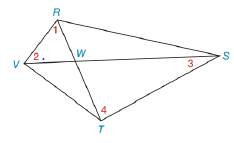Chapter 2.5, Problem 29E### Elementary Geometry for College St...

6th Edition
Daniel C. Alexander + 1 other
ISBN: 9781285195698

#### Solutions

Chapter
Section### Elementary Geometry for College St...

6th Edition
Daniel C. Alexander + 1 other
ISBN: 9781285195698
Textbook Problem
1 views

# Given: Quadrilateral RSTV with diagonals R T ¯ and S V ¯ intersecting at WProve: m ∠ 1 + m ∠ 2 = m ∠ 3 + m ∠ 4To determine

To prove:

The statement m1+m2=m3+m4.

Explanation

Given:

The following figure shows the given quadrilateral.

Approach:

(1) If two lines intersect each other, then vertical angles will be equal.

(2) The sum of three angles in any triangle will be 180°.

Calculation:

The proof is as follows.

### Still sussing out bartleby?

Check out a sample textbook solution.

See a sample solution

#### The Solution to Your Study Problems

Bartleby provides explanations to thousands of textbook problems written by our experts, many with advanced degrees!

Get Started

#### Evaluate the integrals in Problems 1-32. Identify the formula used. 13.

Mathematical Applications for the Management, Life, and Social Sciences

#### True or False: If f(x) = g(x) for all x then f(x) = g(x).

Study Guide for Stewart's Single Variable Calculus: Early Transcendentals, 8th

#### In Exercises 116, determine whether the argument is valid. pq~pq

Finite Mathematics for the Managerial, Life, and Social Sciences

#### Graph each function. fx=log1/4x

College Algebra (MindTap Course List)